Next: Bibliography Up: hoffmann_diss Previous: D. Database of hand-written

# E. Notation and Symbols

Some mathematical notations are used throughout this book: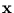a vector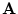a matrix
xi
component of the vectoraij
component of the matrix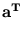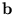scalar product of the vectors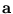and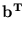matrix with components aibj (direct product)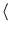x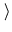expectation value of a random variable x
{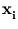}
set of vectors with index i
p(| j)
probability ofgiven the condition j (conditional probability)

The meaning of often used symbols:

t
time (discrete)
St
sensory state at time t
Mt
motor command at time t
IR
set of all real numbers
n
number of training patterns
d
dimension of training patterns
m
number of units in a mixture, or for kernel PCA, the number of points in a reduced set
q
number of principal components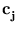code-book vector or the center of the unit j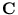covariance matrix of a data distribution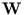d×q matrix containing the principal components as columns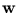a principal component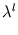eigenvalue belonging to the principal component l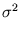residual variance per dimension.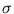is also used as the width of a Gaussian function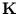kernel matrix

In this book, the following abbreviations appear:

PCA
principal component analysis (or analyzer)
MLP
multi-layer perceptron
RNN
recurrent neural network
SOM
self-organizing map
PSOM
parametrized self-organizing map
NGPCA
neural gas extended to principal component analysis
MPPCA
mixture of probabilistic principal component analyzers
RRLSA
robust recursive least square algorithmNext: Bibliography Up: hoffmann_diss Previous: D. Database of hand-written
Heiko Hoffmann
2005-03-22